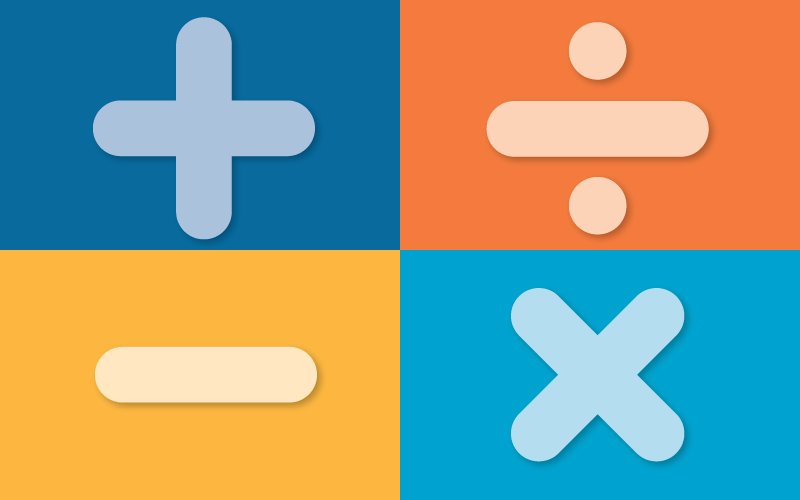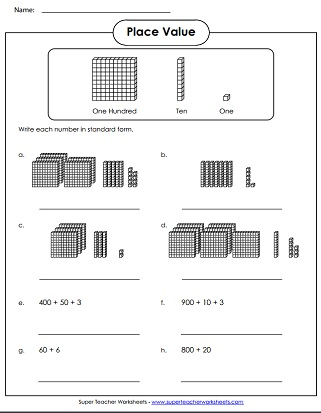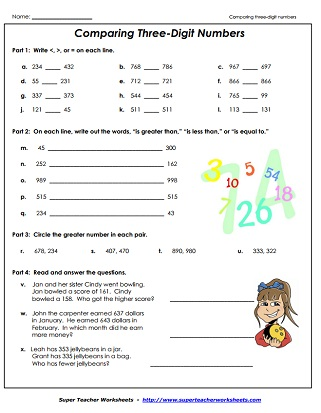# Place Value 3-Digit Numbers

These games and printable worksheets can be used to help students learn about Place Value of 3-digit numbers. Below you'll find links to PDF teaching resources for: expanded form, ordering numbers, reading numbers, counting base-10 blocks, and determining the values of underlined digits.Place Value
What 3-digit numbers are represented by the base-10 place value blocks?
Students line up in front of the classroom and hold up signs with digits on them. They rearrange themselves to make different numbers.
Print and cut the puzzle pieces to match place value blocks to a number. This game goes up to the hundreds place.
Read each 3-digit number and tell how many hundreds, tens, and ones are in each number.
This whole-class "I have, Who has" card game has pictures of place value blocks. Includes 30 cards, instructions, and an answer key.
Write each word name in standard form. Then write each standard form number in word name form. Includes numbers up to 999.
The ancient Egyptian number system is similar to our own. In this lesson, students will learn to read and write three-digit numbers and their Ancient Egyptian equivalents.
Determine which 3-digit number is shown on each abacus picture
Place base 10 blocks on the place value mat to learn about 3-digit numbers.
Cut out the number strips and sort them into groups of equivalent numbers. Also tell which are written in standard form, which are word names, and which are written in expanded form.
Make the smallest and largest number possible by rearranging the given digits.
Students must read the given number and tell what comes before and after.  They also have to add ten and subtract ten.
Draw straight lines to match each three-digit number with its word name.
Make your own three digit numbers with this place value chart.
Printable base-10 PV blocks. This file includes thousand cubes, hundred flats, ten sticks, and ones cubes.
3-Digit Numbers
Students read the 3-digit numbers aloud. Includes 2 score sheets for teachers.
Say the names of the 3-digit numbers.  Includes a parent read aloud and a quiz.
Print on card stock and assemble the number wheel. Students spin the wheel and read the numbers to a teacher, parent, or friend.
Special Number
Show the set of place value blocks represents 349. Write 349 in expanded form. State how many 100s, 10s, and 1s are in the special number.
Write 506 in expanded form. Then tell the value of each digit. Complete the number line to tell what comes before and after 506.
Use "greater than" and "less than" symbols to compare 123 to other numbers. Complete the number line. Tell how many hundreds, tens, and ones.
Determine whether the number 780 is odd or even. Choose the set of blocks that represent 780. How much is 10 mor and 10 less than 780?
Then tell what 100 more and 100 less than 467 equals. Tell how many 100s, 10s, and 1s are in 467. Complete the number line to show numbers that come after and before 467.
Ordering Numbers
Cut apart the three-digit number cards and sort them in order from least to greatest. This file includes 5 different card sets.
Students must examine four triple-digit numbers and rewrite them in order, from smallest value to largest value.
Each of the adorable dancing pigs has a 3-digit number.  Cut, sort, and glue the pigs in order from least to greatest.
Comparing Numbers
Students take turns rolling a die three times to make a triple-digit number. Then they compare their numbers using the symbols: <, >, and =.
Students use scissors to cut out the "greater" and "less" alligator pictures. Then they paste the gator pics in the correct boxes.
Here's another cutting and pasting activity. Like the activity above, pupils are gluing the alligators in position to compare numbers. They also write out the answer with words.
Use words and math notation to compare pairs of numbers.  This worksheet includes some word problems too.  (example: 324 < 342)
Compare the values of the numbers shown. (example: 562 > 545)
On these task cards, students use greater than, less than, or equal to symbols to compare 3-digit numbers. These cards work well for learning centers, Scoot games, classroom scavenger hunts, and small group instruction.
Cut outthese number, symbol, and phrase cards. Use them for small group instruction, learning centers, or math games.
4-Digit Place Value

Worksheet Images